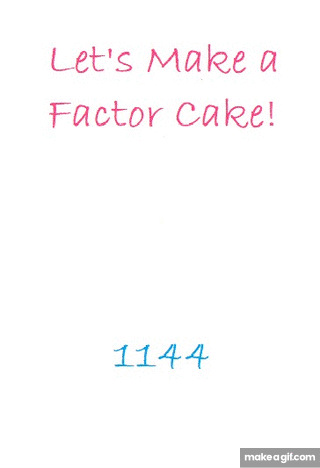# 1144 Let’s Make a Factor Cake!

Occasionally, I’ll put a factor cake on this blog, but until today, I haven’t really shown you how to MAKE a factor cake. I made this simple gif to show you how to make a factor cake from start to finish. You can watch it over and over again if you like. Just repeatedly divide by the smallest prime number divisor.make science GIFs like this at MakeaGif

Here’s more about the number 1144:

• 1144 is a composite number.
• Prime factorization: 1144 = 2 × 2 × 2 × 11 × 13, which can be written 1144 = 2³ × 11 × 13
• The exponents in the prime factorization are 3, 1, and 1. Adding one to each and multiplying we get (3 + 1)(1 + 1)(1 + 1) = 4 × 2 × 2 = 16. Therefore 1144 has exactly 16 factors.
• Factors of 1144: 1, 2, 4, 8, 11, 13, 22, 26, 44, 52, 88, 104, 143, 286, 572, 1144
• Factor pairs: 1144 = 1 × 1144, 2 × 572, 4 × 286, 8 × 143, 11 × 104, 13 × 88, 22 × 52, or 26 × 44
• Taking the factor pair with the largest square number factor, we get √1144 = (√4)(√286) = 2√286 ≈ 33.823071144 is obviously divisible by 11, but it isn’t so obvious that 1144 is the sum of the twenty-two prime numbers from 11 to 101.

1144 is the hypotenuse of a Pythagorean triple:
440-1056-1144 which is (5-12-13) times 88

1144 is palindrome 3223 in BASE 7 because 3(7³) + 2(7²) + 2(7) +3(1) = 1144

This site uses Akismet to reduce spam. Learn how your comment data is processed.